Get instant live expert help with Excel or Google Sheets“My Excelchat expert helped me in less than 20 minutes, saving me what would have been 5 hours of work!”

#### Post your problem and you’ll get Expert help in seconds.

Your message must be at least 40 characters
Our professional Expert are available now. Your privacy is guaranteed.

# Filter values in array formula

Excel allows us to filter values in an array with the IF, ISNUMBER and MATCH functions. This step by step tutorial will assist all levels of Excel users in filtering in or out some specific values in a data set.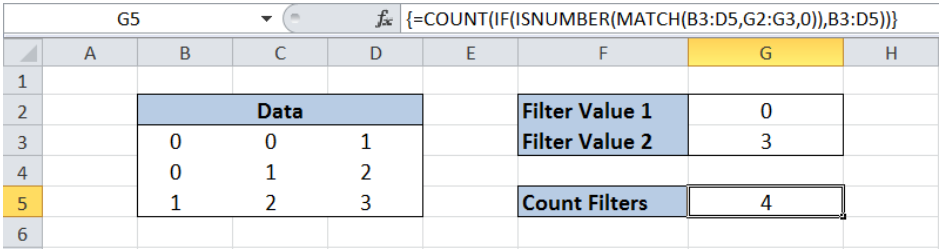Figure 1. Final result: Filter values in array

Final formula: `=COUNT(IF(ISNUMBER(MATCH(B3:D5,G2:G3,0)),B3:D5))`

## Syntax of the IF function

IF function evaluates a given logical test and returns a TRUE or a FALSE.

### Syntax

`=IF(logical_test, [value_if_true], [value_if_false])`

• The arguments “value_if_true” and “value_if_false” are optional.  If left blank, the function will return TRUE if the logical test is met, and FALSE if otherwise.

## Syntax of the ISNUMBER function

The ISNUMBER function tests whether a value is a number and returns TRUE; FALSE if otherwise

### Syntax

`=ISNUMBER(value)`

• Value – any value that we want to test if a number or not

## Syntax of the MATCH function

MATCH function is used to search for a specific value in a range of cells.  It returns the #N/A error value if the search is unsuccessful.

### Syntax

`=MATCH(lookup_value, lookup_array, [match_type])`

The parameters are:

• lookup_value – a value which we want to find in the lookup_array
• lookup_array – the range of cells containing the value we want to match
• [match_type] optional; the type of match; if omitted, the default value is 1; We use 0 to find an exact match

## Setting up Our Data

Our data shows a 3×3 table with values 0, 1, 2 and 3.  In cells G2 and G3, we specify the values we want to filter, which are numbers 0 and 3.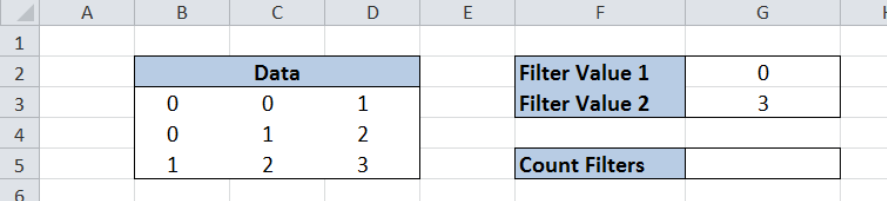Figure 2. Sample data to filter values in array

## Filter Values in Array

We want to filter the values 0 and 3 in our data.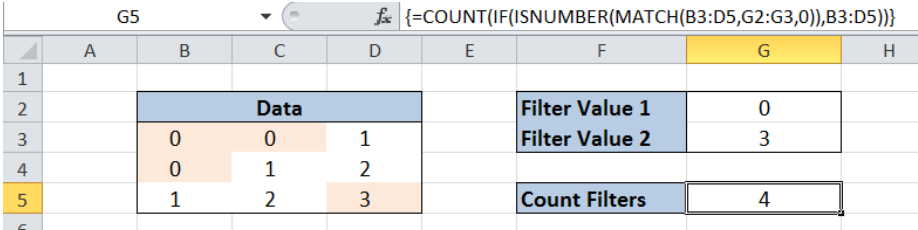Figure 3. Entering the formula to filter values in array

To filter values in an array, we follow these steps:

Step 1.  Select cell G5.

Step 2.  Enter the formula: `=COUNT(IF(ISNUMBER(MATCH(B3:D5,G2:G3,0)),B3:D5))`

Step 3.  Press Ctrl + Shift + ENTER because this is an array formula.

First, the formula matches our data with the filter values 0 and 3 through the MATCH function “`MATCH(B3:D5,G2:G3,0)`”. The ISNUMBER evaluates the matched values and returns TRUE if there is a match. Otherwise it returns FALSE.

The result is an array with these values:  `{TRUE,TRUE,FALSE;TRUE,FALSE,FALSE;FALSE,FALSE,TRUE}`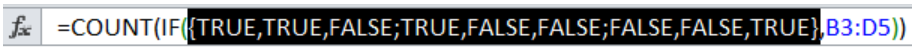Figure 4. Resulting array after the matched values are evaluated if a number or not

The COUNT and IF functions return the count for the filtered values. As a final result, the value in cell G5 is 4, which is the count of all values 0 and 3 in our data.

## Exclude Filters in Array

There are instances when we want to exclude the filtered values from our data.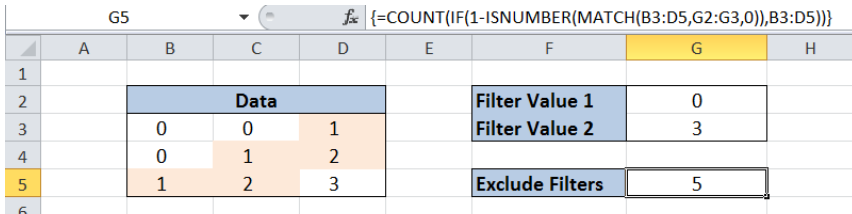Figure 5. Entering the formula to exclude filtered values in array

To exclude filtered values in an array, we follow these steps:

Step 1.  Select cell G5.

Step 2.  Enter the formula: `=COUNT(IF(1-ISNUMBER(MATCH(B3:D5,G2:G3,0)),B3:D5))`

Step 3.  Press Ctrl + Shift + ENTER .

The result in cell G5 is the reverse of the result in our previous example. Out of the 9 data points, we excluded the filtered values 0 and 3, and came up with 5 values.

Most of the time, the problem you will need to solve will be more complex than a simple application of a formula or function. If you want to save hours of research and frustration, try our live Excelchat service! Our Excel Experts are available 24/7 to answer any Excel question you may have. We guarantee a connection within 30 seconds and a customized solution within 20 minutes.

### Did this post not answer your question? Get a solution from connecting with the expert.Another blog reader asked this question today on Excelchat:
Solution examplesI need to find out if the 2018 yearly sales goals were met if the yearly sales were \$25,000 or more using an IF logical function and to set the formula to return a value of YES if met, and NO if not
Solved by A. A. in 18 minsI want to display a range of cells if they meet a specific criteria. I want to display a name IF it does not equal one of three options AND there are any numbers contained in a range of cells
Solved by G. F. in 60 minsï?·In cell G1enter Discount, ï?·In cell H1enter DiscountPercent,ï?·In cell I1enter DiscountCost,ï?·In cell J1enter TotalCost, andï?·In cell K1enter Paymentas column headings.
Solved by K. E. in 40 minsHow do you get excel to ignore a formula until cell contains a value i.e.(if B1 is 1000, don't subtract C1 from B1 for a sum in C2 (resulting in -1000), until a value is first put in C1. ANOTHER EXAMPLE Don't add the sum of D5 and D6 in D7 if D5 and D6 are blank, preventing D7 (and E7 and G7...) from showing up as 0.00 ). I would like totals to remain blank and not the whole row showing 0.00 until a number is placed to complete the formula
Solved by V. J. in 28 minsNeed help with a formula, I'd like it to be something similar to '' =IF(AB2=AC2,"Matches","No Match") '' but instead of if they 100% match I want a 5% variable for example, if Cell A1 contains 12:00 and Cell B1 contains 11:59 I want it to class as matching.
Solved by Z. J. in 13 mins## Subscribe to Excelchat.coAnother blog reader asked this question today on Excelchat: